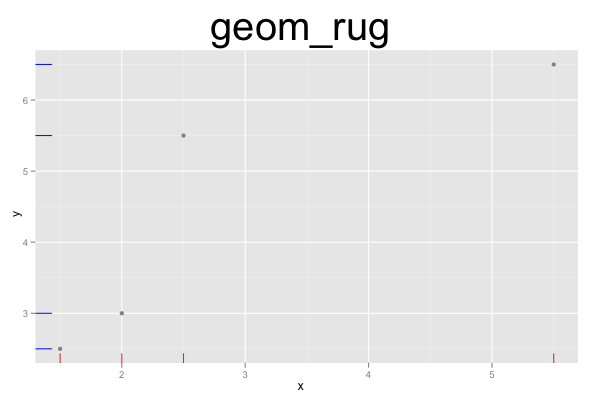## ggplot2 Quick Reference: geom_rug

A geom that draws a rug along the x and/or y axis.

Default statistic: stat_identity

### Parameters

• x - to draw a rug along the x-axis (one short line for each x value)
• y - to draw a rug along the y-axis (one short line for each y value)
• size - (default: 0.5) line width of the rug's lines
• linetype - (default: 1=solid) line type of the rug's lines
• color - (default: "black") color of the rug's lines
• alpha - (default: 1=opaque) transparency of the rug's lines

Note that x and y can be used at the same time to draw rugs along both axes.

### Example

The following example plot contains three layers. The first layer contains a horizontal rug (in red), the second layer contains a vertical rug (in blue), and the third layer shows the underlying points. The two rugs could also be drawn in the same layer, but then the corresponding x and y lines would have to have the same color.```d=data.frame(x=c(1.5, 2, 5.5, 2.5), y=c(2.5, 3, 6.5, 5.5))
ggplot() +
geom_rug(data=d, mapping=aes(x=x), color="red") +
geom_rug(data=d, mapping=aes(y=y), color="blue") +
geom_point(data=d, mapping=aes(x=x, y=y), color="grey50") +
opts(title="geom_rug", plot.title=theme_text(size=40, vjust=1.5))```